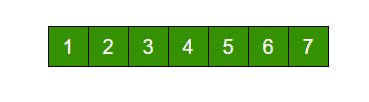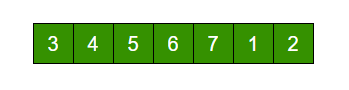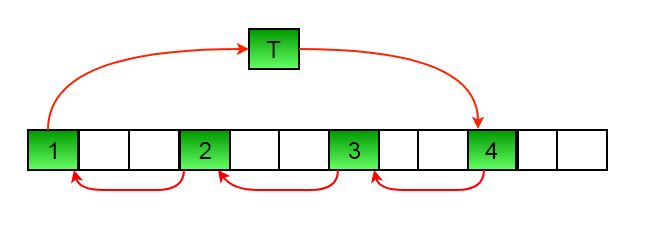GeeksforGeeks App
Open AppBrowser
Continue

# C# Program for Program for array rotation

Write a function rotate(ar[], d, n) that rotates arr[] of size n by d elements.Rotation of the above array by 2 will make arrayMETHOD 1 (Using temp array)

```Input arr[] = [1, 2, 3, 4, 5, 6, 7], d = 2, n =7
1) Store the first d elements in a temp array
temp[] = [1, 2]
2) Shift rest of the arr[]
arr[] = [3, 4, 5, 6, 7, 6, 7]
3) Store back the d elements
arr[] = [3, 4, 5, 6, 7, 1, 2]```

Time complexity : O(n)
Auxiliary Space : O(d)

METHOD 2 (Rotate one by one)

```leftRotate(arr[], d, n)
start
For i = 0 to i < d
Left rotate all elements of arr[] by one
end```

To rotate by one, store arr in a temporary variable temp, move arr to arr, arr to arr …and finally temp to arr[n-1]
Let us take the same example arr[] = [1, 2, 3, 4, 5, 6, 7], d = 2
Rotate arr[] by one 2 times
We get [2, 3, 4, 5, 6, 7, 1] after first rotation and [ 3, 4, 5, 6, 7, 1, 2] after second rotation.
Below is the implementation of the above approach :

## C#

 `// C# program for array rotation``using` `System;` `class` `GFG {``    ``/* Function to left rotate arr[]``    ``of size n by d*/``    ``static` `void` `leftRotate(``int``[] arr, ``int` `d,``                           ``int` `n)``    ``{``        ``for` `(``int` `i = 0; i < d; i++)``            ``leftRotatebyOne(arr, n);``    ``}` `    ``static` `void` `leftRotatebyOne(``int``[] arr, ``int` `n)``    ``{``        ``int` `i, temp = arr;``        ``for` `(i = 0; i < n - 1; i++)``            ``arr[i] = arr[i + 1];` `        ``arr[n-1] = temp;``    ``}` `    ``/* utility function to print an array */``    ``static` `void` `printArray(``int``[] arr, ``int` `size)``    ``{``        ``for` `(``int` `i = 0; i < size; i++)``            ``Console.Write(arr[i] + ``" "``);``    ``}` `    ``// Driver code``    ``public` `static` `void` `Main()``    ``{``        ``int``[] arr = { 1, 2, 3, 4, 5, 6, 7 };``        ``leftRotate(arr, 2, 7);``        ``printArray(arr, 7);``    ``}``}` `// This code is contributed by Sam007`

Output :

`3 4 5 6 7 1 2 `

Time complexity : O(n * d)
Auxiliary Space : O(1)
METHOD 3 (A Juggling Algorithm)
This is an extension of method 2. Instead of moving one by one, divide the array in different sets
where number of sets is equal to GCD of n and d and move the elements within sets.
If GCD is 1 as is for the above example array (n = 7 and d =2), then elements will be moved within one set only, we just start with temp = arr and keep moving arr[I+d] to arr[I] and finally store temp at the right place.
Here is an example for n =12 and d = 3. GCD is 3 and

```Let arr[] be {1, 2, 3, 4, 5, 6, 7, 8, 9, 10, 11, 12}

a) Elements are first moved in first set – (See below
diagram for this movement)``````          arr[] after this step --> {4 2 3 7 5 6 10 8 9 1 11 12}

b)    Then in second set.
arr[] after this step --> {4 5 3 7 8 6 10 11 9 1 2 12}

c)    Finally in third set.
arr[] after this step --> {4 5 6 7 8 9 10 11 12 1 2 3}```

Below is the implementation of the above approach :

## C#

 `// C# program for array rotation``using` `System;` `class` `GFG {``    ``/* Function to left rotate arr[]``    ``of size n by d*/``    ``static` `void` `leftRotate(``int``[] arr, ``int` `d,``                           ``int` `n)``    ``{``        ``int` `i, j, k, temp;``        ``/* To handle if d >= n */``        ``d = d % n;``        ``int` `g_c_d = gcd(d, n);``        ``for` `(i = 0; i < g_c_d; i++) {``            ``/* move i-th values of blocks */``            ``temp = arr[i];``            ``j = i;``            ``while` `(``true``) {``                ``k = j + d;``                ``if` `(k >= n)``                    ``k = k - n;``                ``if` `(k == i)``                    ``break``;``                ``arr[j] = arr[k];``                ``j = k;``            ``}``            ``arr[j] = temp;``        ``}``    ``}` `    ``/*UTILITY FUNCTIONS*/``    ``/* Function to print an array */``    ``static` `void` `printArray(``int``[] arr, ``int` `size)``    ``{``        ``for` `(``int` `i = 0; i < size; i++)``            ``Console.Write(arr[i] + ``" "``);``    ``}` `    ``/* Function to get gcd of a and b*/``    ``static` `int` `gcd(``int` `a, ``int` `b)``    ``{``        ``if` `(b == 0)``            ``return` `a;``        ``else``            ``return` `gcd(b, a % b);``    ``}` `    ``// Driver code``    ``public` `static` `void` `Main()``    ``{``        ``int``[] arr = { 1, 2, 3, 4, 5, 6, 7 };``        ``leftRotate(arr, 2, 7);``        ``printArray(arr, 7);``    ``}``}` `// This code is contributed by Sam007`

Output :

`3 4 5 6 7 1 2 `

Time complexity : O(n)
Auxiliary Space : O(1)

Please see following posts for other methods of array rotation:
Block swap algorithm for array rotation
Reversal algorithm for array rotation